# UMB Practice for Placement Test B

The questions below will review the areas of mathematics that are covered in Test B. These areas are:

* rules for exponents (integral and negative)       * absolute value

* square roots                                                       * manipulating literal fractions having letters

* factoring                                                            * solving simultaneous equations

* word problems                                                  * lines and points graphed on a plane

* inequalities                                                        * solving quadratic equations

You should try to review these questions (and take Test B) if you have taken two years of high school algebra or have taken one or two semesters of College Algebra. Successful completion of Test B will place you into Statistics (Math 125) and PreCalculus (Math 129 or 130). After submitting the review questions you will receive an analysis of what courses you would be  eligible to take if this review had been Placement Test B instead of Practice for Placement Test B. Answers will be given after clicking the submit button below. In addition, a link will be provided to an interactive PreCalculus review which might be helpful for any additional review.

There are 43 questions in two parts.

Click the Submit button at the bottom of the test when you are finished.

Please forward any comments, criticisms, suggestions to: Michael Greeley

Part One
In problems 1 - 3, find the numerical value when a = -3 and b = 2:

 1 a2- b2 a+b -a4
 -86 -76 -21 76 86

2.    (-b)4 + 3a + 5a2 - 2a3

 -16 -16 74 101 106

3.    a - |a + b| + |a - b|
 -3 1 3 7 15

In Problems 4 and 5, simplify the expression as much as possible: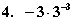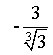-1
 - 19
 19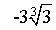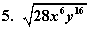14x6y16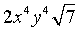14x3y8 2x3y8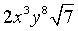In Problems 6 and 7, solve for x:

 6 5(x + 8)7 - 3 = 12 x + 4
 1 12
3 6 -8
 - 283

 7 1x + 1x + 3 = 53x

 1 6 -1 12 15

In Problems 8 - 13, perform the indicated operations and simplify: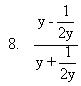-1   3   2y
 2y2-12y2+1

 2y2+12y2-1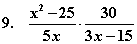103
2(x+5)
 - 103

 2(x + 5)x

 2(x - 5)x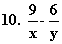3x + y
 3x - y
 3xy
 9y - 6xxy
 9x - 6yxy

 11 (a3b5)2c3a4b10c4
 a2c
 a6c
 a2b10c

 a6b10c

 a10b20c7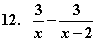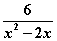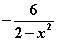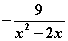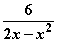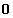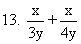7x12y
 7x12y2
7xy
 2x7y
12xy
 14. Find an expression equivalent to - 4a - bc - 5d

 - b - 4a5d - c b - 4a5d - c - b - 4ac - 5d -(b - 4a)5d - c -4a - bc - 5d

15.  Solve for w:  b(3 + w) - 1 = b - 13w

 1 + 13b2 + b
 1 - 2b13 + b
 1 + 13bb - 2
 1 - 13b4 + b
 1 - 3b2 + b

16.  Find all real values of x such that 48x2 - 33 = 0.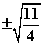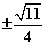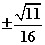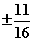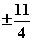17. Given that q3 = 11 and x15 = 121, express x in terms of q.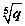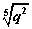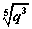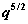q5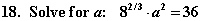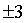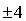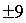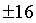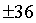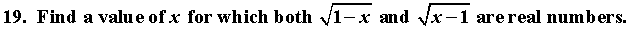-2 -1 0 1 2

20.  If a is a real number such that a3 = a2   , which of the following must be true about a?

 a is positive a is negative a = -1 a = 0 or a = 1 a is between -1 and 0

In Problems 21 and 22,  Solve for x.

21. 2x + 6 > 3x - 4
 x > -10 x < -10 x < 10 x > 2 x < 2

22.  |4 - x| < 4

 x < 0 x < 8 0 < x < 8 x > 0 x > 8

23.  Which of the following is a solution of 6x2 = 5x + 50?

 -2/5 -10/3 3 10 none of these.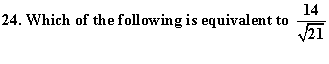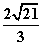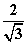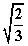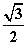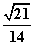25.  Find all ordered pairs (x,y) satisfying |x| + |y| = 0.

 (1,-1) and (-1,1) (0,0) and (1,-1) (0,0) (0,0) and (1,0) there are none

26.  Find the solution (x,y) to these simultaneous equations:

3x + 4y = 17

2x -  y   = 4

 (5, 1/2) (2,3) (11,-4) (3,2) (0,17/4)

27.  Find all solutions (x,y) satisfying these simultaneous equations:

x + y = 8

xy = 0

 (0,8) and (8,0) (0,8) and (0,-8) (0,0) (0,0) and (4,4) (2,6), (4,4) and (6,2)

28.  Find the equation of the straight line passing through the points (7,19) and (9,23).

 y = 2x + 19 y = 3x - 23 y = 4x - 19 y = 2x + 5 y = -2x +33

29.  Which statement is true about the slope m of any straight line perpendicular to the graph

of 3x + y = 129?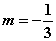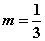m = -3 m = 3 none of those

30.  Find the value of y when x = -1 for the following graph: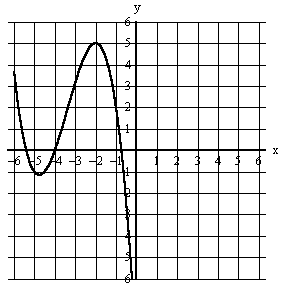-3 -2 -1 1 2

31.  What is true of lines L and M, graphed here?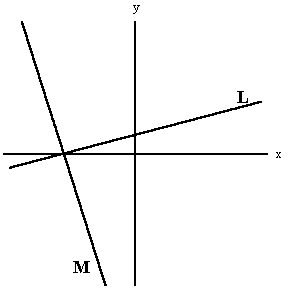They have the same slope. Both have positive slopes. Both have negative slopes. They have the same x-intercept They have the same y-intercept

32.  Which diagram contains the graphs of y = -x2 + 2x and x = 2?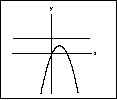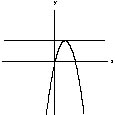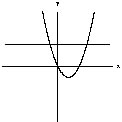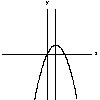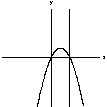Part Two

33.  Given that it is not true that all the students in a math class of seventeen students are Irish, which of the following statements follow logically from the given?

 None of the students are Irish. Some of the students are Irish. At least one of the students is not Irish. At least two of the students are not Irish. None of the above four statements follows logically from the given.

34.  Suppose that at most seventeen people in the world really understand Steven Hawking's theories of the origin of the universe. Further, suppose that at least six of them live in England, at most two of them live in Wales, at least three of them live in France and at least four of them live outside of France.

Although the exact number of them who live outside of Great Britain is actually indeterminate without further information, there is a smallest range in which that number must certainly lie. Find that range, assuming that all ranges listed are inclusive of the endpoints.

(Great Britain consists of England, Scotland and Wales.)

 0 to 11 2 to 11 3 to 11 4 to 17 7 to 15

35.  You have 20 identical black socks and 20 identical white socks in a dresser drawer. Assuming that it is too dark to see and all socks feel the same, how many socks must you pick to make sure that you get a pair?

 2 3 4 20 21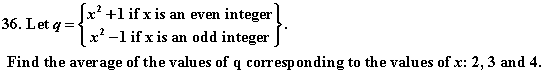5 9 1/3 10 17 30

37.  Photocopies of a document cost 5 cents each. There is a discount of 20% for copies eleven through thirty and a discount of 40% for copies in excess of thirty. Find the cost of photocopying 100 copies of a document.

 \$3.00 \$3.40 \$3.60 \$4.30 \$5.00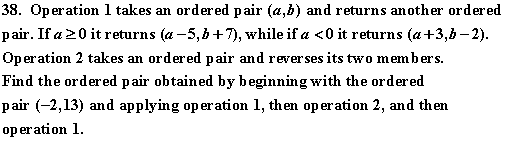(13,-2) (23,-9) (5,8) (6,8) (1,11)

39.  Take a number x. Triple it; then subtract 1 from the result; next square this result; and finally add the original number to the last result.

Express in terms of x the result of the above four-step process.

 4x2 - x - 1 4x2 - 7x + 4 8x2 - 2 9x2 - 3x 9x2 - 5x + 1

40.  Ball-point pens cost 39 cents each. Pencils cost 7 cents each. Assuming that you have at most \$4.92 to spend and you must buy exactly 20 items, find the smallest number of pencils you can buy.

 0 8 9 11 none of those
 41. Suppose     x = 23 .     Evaluate     x-3

 - 278

 - 69

 - 827

 96

 278

42.  A side of square B is 3 less than a side of square A. If you double the area of square B and add 2, your result equals the area of square A.

Find the length of a side of square A.

 2 3 7 10 12

43.  A rectangular rock garden 19 yards long and 10 yards wide is surrounded by a stone path of uniform width. The outer boundaries of the path form a rectangle concentric with the garden.

If the path were made 1 yard wider, its area would increase by 118 square yards. Find the width of the path.

 7 yards 8 yards 10.625 yards 22.5 yards 56 yards